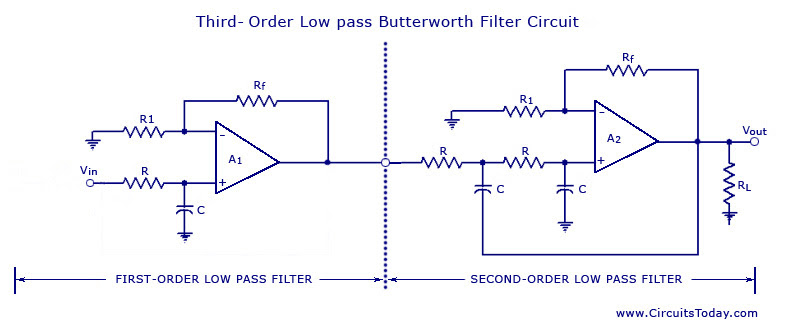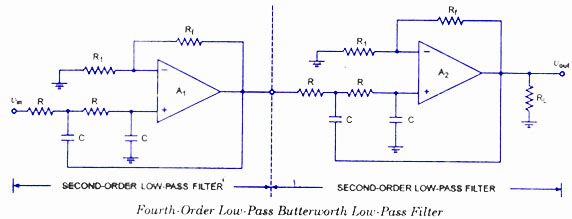## Audio

[Audio][slideshow]

## Higher Order Filters

From the discussion made so far on the filters, it may be concluded that in the stopband the gain of the filter changes at the rate of 20 db/decade for first-order filters and 40 db/decade for second-order filters. This means that as the order of the filter is increased, the actual stopband response of the filter approaches its ideal stopband characteristics. In general, a third-order filter produces 60 db/decade, a fourth-order filter produces 80 db/decade and so on.

Higher-order filters, such as third, fourth, fifth, and so on, are built simply by using the first and second-order filters.

The simplest way to build a third-order low-pass filter is by cascading a first order filter with a second-order. Similarly a fourth-order low-pass filter can be formed by cascading two second-order low-pass filters. Although there is no limit to the order of the filter that can be formed, as the order of the filter increases, so does its size. Also the accuracy declines, in that the difference between the actual stopband response and the theoretical stopband re­sponse increases with an increase in the order of the filter.A third-order low-pass Butterworth filter is illustrated in figure.

The voltage-gain of the first section is optional, it can be set, whatever is required. The voltage-gain of the second section, however affects the flatness of the overall response. If closed-loop gain is kept 1.586, then the overall gain will be down 6 db (3 db for each section) at the cut-off frequency. By increasing the voltage gain of the second section slightly, cumu­lative loss of voltage gain is offset. By using an advanced mathematical derivation, it can be proved that an Af, of 2 is the critical value required for a maximally flat response.

In this case Rf = R1

When Af = 2, the cut-off frequency is given as

fH = 1 / 2∏RC

where R and C are the resistance and capacitance of each section. At cut-off frequency, the overall voltage gain is down 3 db. Above the cut-off frequency, the voltage gain drops at a rate of 60 db per decade equivalent to 18 db per octave.A fourth-order low-pass Butterworth filter is illustrated in figure. It is formed by cascading two second-order low-pass filters. If Af, of 1.586 is used for both sections, the voltage gain will be down 6 db at the cut-off frequency. By using different gain for each section, we can strike a compromise that produces a maximally flat response. An advanced derivation shows that we need to use Af= 1.152 for the first section and Af = 2.235 for the second section.

Also, the overall filter gain is equal to the product of the individual voltage gains of the filter sections. Hence, the overall gain of a fourth-order filter is 1.152 x 2.235 = 2.575.

In all our Butterworth designs, the cut-off frequency is given as 1 / 2∏RC

As with the first- and second-order filters, the third- and fourth-order high-pass filters are formed by simply interchanging the positions of the frequency determining resistors and capacitors in the corresponding low-pass filters. The high-order filters can be designed by following the procedures outlined for the first- and second-order filters.

Generally, the minimum-order filter required depends on the application specifications. Although a high-order filter than necessary provides a better stopband response, the high-order filter is more complex, occupies more space and is more expensive.

It is worth mentioning here that in all filters, the same resistance and capacitance values are used in the bypass or R-C networks, a definite convenience in selection of components and ease of construction. This fixes the overall gain of the high-order filters. Furthermore, the 3-db cut-off frequency is always the same and is equal to 1/2∏RC

[Lights][grids]